# B-field for a half-infinitely long wire

Callumnc1
Homework Statement:
Relevant Equations:
For this problem,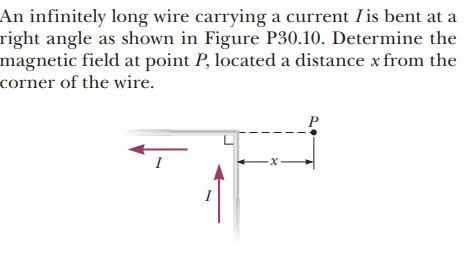The solution is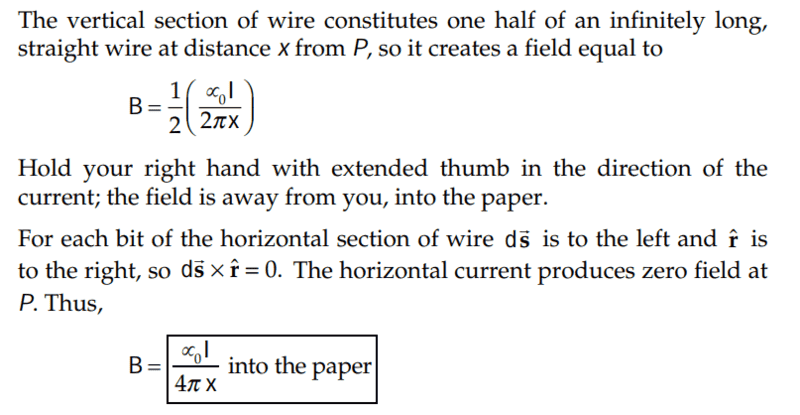However, I would like to understand how to solve this using Biot–Savart Law.

So far my working is:

## \vec {dB} = \frac {\mu_0Ids\sin\theta} {4\pi r^2}##

However, I'm not sure what to do about the ## r^2 ## since the wire is infinitely long. I am thinking about having the limits of integration to be ## \theta_1 = 0 ## and ## \theta_2 = \frac {\pi} {2} ##

Many thanks!

Homework Helper
Gold Member
2022 Award
.So far my working is:

## \vec {dB} = \frac {\mu_0Ids\sin\theta} {4\pi r^2}##
Knowing a formula is of no value unless you know what all the variables mean and in what context the formula applies.
Please state what those variables mean here. From that you should be able to answer your question.

•anuttarasammyak, Callumnc1 and PeroK
Gold Member
As your LHS is vector, RHS should be vector also. Check it out.

•Callumnc1
Callumnc1
Knowing a formula is of no value unless you know what all the variables mean and in what context the formula applies.
Please state what those variables mean here. From that you should be able to answer your question.

##\mu_0## is the magnetic permeability of free space
##I## is the current in the vertical wire
## ds ## is the length element of the wire
## r^2 ## is the distance from each ##ds## to point ##P##

I think the thing that was tripping me up was that I thinking that we could call the infinite length to be ##a## which would mean that ##\tan\theta = \frac {x} {a} ## however this would be zero since ## a \rightarrow \infty ## then ##\tan \theta \rightarrow 0 ##. I was then going to solve for ##\theta## and have limits of integration to be angles ## \theta_1 = 0 ## and ## \theta_2 = \frac {\pi} {2} ##. However now I think I should get ## r ## in terms of ## \sin\theta ##. I will try to solve that now. Is my reasoning correct so far?

Last edited:
•TSny
Callumnc1
As your LHS is vector, RHS should be vector also. Check it out.
Thanks @anuttarasammyak ! Sorry both sides should have ##-\hat k## as the direction.

Homework Helper
Gold Member
2022 Award
I should get ## r ## in terms of ## \sin\theta ##.
Yes.

•Callumnc1
Alex Schaller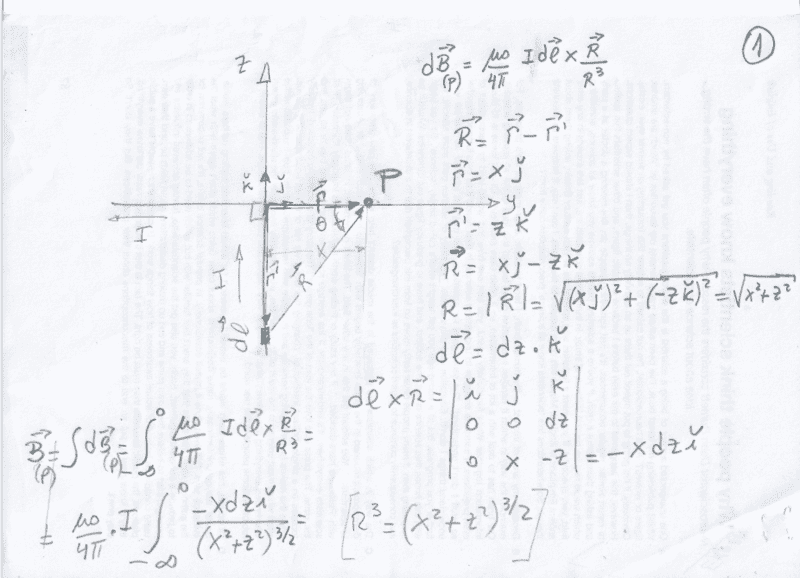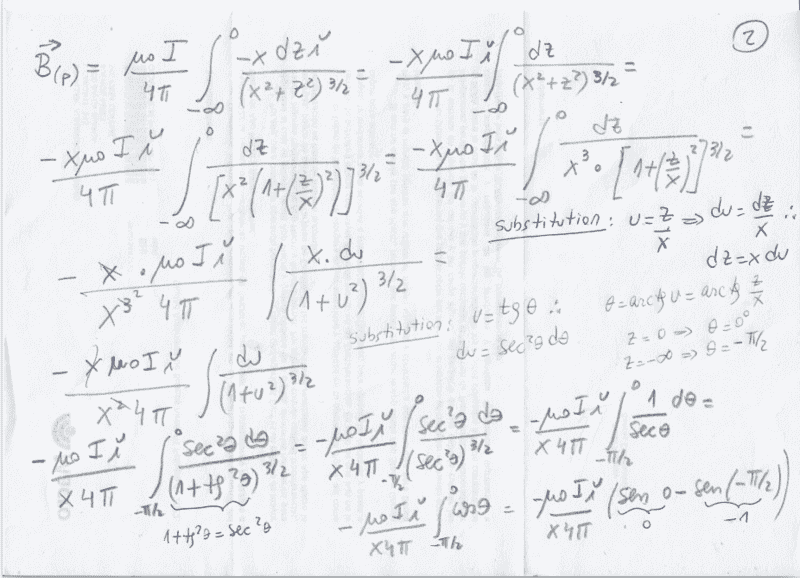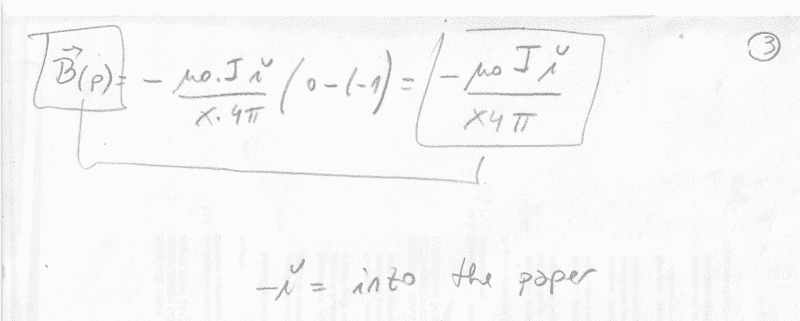•Callumnc1
Callumnc1
Callumnc1
Alex Schaller
Thank you for your solution @Alex Schaller! :)
You are welcome

•Callumnc1